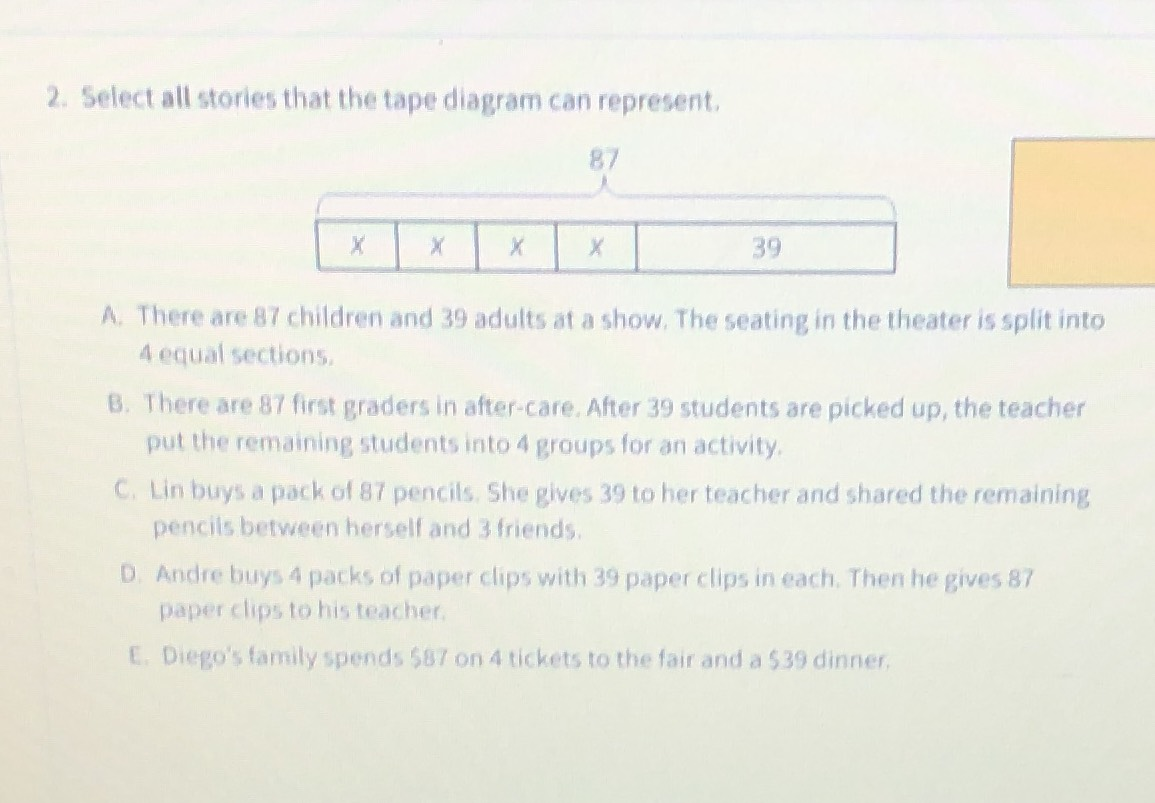### ¿Todavía tienes preguntas de matemáticas?

Pregunte a nuestros tutores expertos
Algebra
Pregunta2. Select all stories that the tape diagram can represent.

A. There are $$87$$ children and $$39$$ adults at a show. The seating in the theater is split into

$$4$$ equal sections.

B. There are $$87$$ first graders in after-care. After $$39$$ students are picked up, the teacher put the remaining students into $$4$$ groups for an activity.

C. Lin buys a pack of $$87$$ pencils. She gives $$39$$ to her teacher and shared the remaining pencils between herself and $$3$$ friends.

D. Andre buys $$4$$ packs of paper clips with $$39$$ paper clips in each. Then he gives $$87$$ paper clips to his teacher.

E. Diego's family spends $$587$$ on $$4$$ tickets to the fair and a $$\ 39$$ dinner.